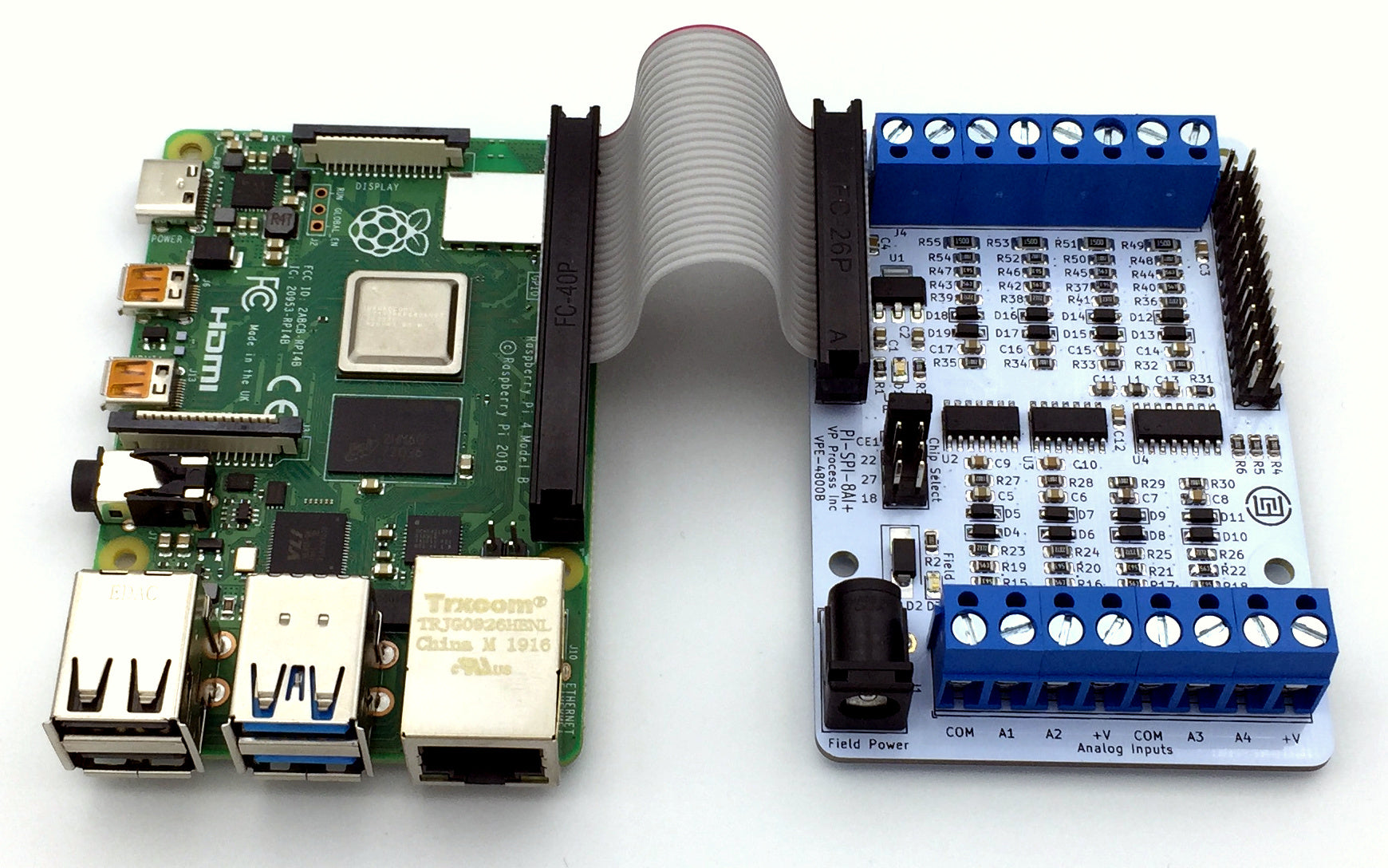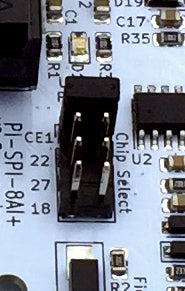# PI-SPI-8AI+ Raspberry Pi with 32 Channels of Analog InputsThe PI-SPI-8AI+ Analog Input Module has been designed with 4 Chip Selects. This allows for up to 32 analog inputs to one Raspberry when using the PI-SPI series.Each input can be either:

0 to 20 mA (4-20 mA)
0 to 5 VDC (full scale 6.6 VDC)
0 to 10 VDC (fill scale 0 to 12 VDC)
10K Thermistor for Temperature  sensing (operating range – 40 to + 85 Deg C)

To read up to 32 analog inputs, there are two requirements:

1. libwidgetlords library version 2.0 (or higher) must be installed
2. The /boot/config.txt file MUST have this statement at the end of the file:

dtoverlay=pi-spi:extra_cs=true

Please note: only one dtoverlay from our library should be enabled at one time.

The four chip selects are shown here:Only 1 jumper should be installed at a time.

Typically, the jumpers are installed as follows:

Jumper CE1 = PI-SPI-8AI+ Module 1 = Analog Inputs 1 thru 8
Jumper 22 =   PI-SPI-8AI+ Module 2 = Analog Inputs 9 thru 16
Jumper 27 =   PI-SPI-8AI+ Module 1 = Analog Inputs 17 thru 24
Jumper 18 =   PI-SPI-8AI+ Module 1 = Analog Inputs 25 thru 32

Here is a simple Pyhon script to read 32 Analog Inputs from 4 PI-SPI-8AI+ modules

from time import sleep
from widgetlords.pi_spi import *

init()

input_module_1 = Mod8AI(0)
input_module_2 = Mod8AI(1)
input_module_3 = Mod8AI(2)
input_module_4 = Mod8AI(3)

while True:

print("PI-SPI-8AI Module 1")
for x in range(8):
print("Input ", x," = ", input_module_1.read_single(x), " AD Counts")

print("")

print("PI-SPI-8AI Module 2")
for x in range(8):
print("Input ", x+8," = ", input_module_2.read_single(x), " AD Counts")

print("")

print("PI-SPI-8AI Module 3")
for x in range(8):
print("Input ", x+16," = ", input_module_3.read_single(x), " AD Counts")

print("")

print("PI-SPI-8AI Module 4")
for x in range(8):
print("Input ", x+24," = ", input_module_4.read_single(x), " AD Counts")

print("")

sleep(1)

``` ```

This block diagram relates to the above sample code